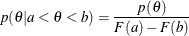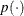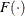# The MCMC Procedure

### Truncation and Censoring

Subsections:

#### Truncated Distributions

To specify a truncated distribution, you can use the LOWER= and/or UPPER= options. Almost all of the standard distributions, including the GENERAL and DGENERAL functions, take these optional truncation arguments. The exceptions are the binary and uniform distributions.

For example, you can specify the following:

prior alpha ~ normal(mean = 0, sd = 1, lower = 3, upper = 45);


or

parms beta;
a = 3; b = 7;
ll = (a + 1) * log(b / beta);
prior beta ~ general(ll, upper = b + 17);


The preceding statements state that if beta is less than b+17, the log of the prior density is ll, as calculated by the equation; otherwise, the log of the prior density is missing—the log of zero.

When the same distribution is applied to multiple parameters in a PRIOR statement, the LOWER= and UPPER= truncations apply to all parameters in that statement. For example, the following statements define a Poisson density for theta and gamma:

parms theta gamma;
lambda = 7;
l1 = theta * log(lambda) - lgamma(1 + theta);
l2 = gamma * log(lambda) - lgamma(1 + gamma);
ll = l1 + l2;
prior theta gamma ~ dgeneral(ll, lower = 1);


The LOWER=1 condition is applied to both theta and gamma, meaning that for the assignment to ll to be meaningful, both theta and gamma have to be greater than 1. If either of the parameters is less than 1, the log of the joint prior density becomes a missing value.

In releases before SAS/STAT 13.1, only three distributions support parameters (or functions of parameters) in the LOWER= and UPPER= options. These are the normal distribution, the GENERAL function, and the DGENERAL function. Appropriate normalizing constants, which are required if the truncations involve model parameters, are not calculated. Starting with SAS/STAT 13.1, PROC MCMC calculates the normalizing constant in all truncated distributions, and you can use parameters in the LOWER= or UPPER= option.

Note that if you use either the GENERAL or DGENERAL function, you must compute the normalizing constant in cases where it is required. A truncated distribution has the probability distributionwhereis the density function andis the cumulative distribution function. In SAS functions,is the probability density function andis the cumulative distribution function. The following example shows how to construct a truncated gamma prior on theta, with SHAPE=3, SCALE=2, LOWER=A, and UPPER=B:

lp = logpdf('gamma', theta, 3, 2)
- log(cdf('gamma', a, 3, 2) - cdf('gamma', b, 3, 2));
prior theta ~ general(lp);


This density specification is different from the following more naive definition, without taking into account the normalizing constant:

lp = logpdf('gamma', theta, 3, 2);
prior theta ~ general(lp, lower=a, upper=b);


If a or b is a parameter, you get very different results from the two formulations.

#### Censoring

There is no built-in mechanism in PROC MCMC that models censoring automatically. You need to construct the density function (using a combination of the LOGPDF, LOGCDF, and LOGSDF functions and IF-ELSE statements) for the censored data.

Suppose you partition the data into four categories: uncensored (with observation x), left censored (with observation xl), right censored (with observation xr), and interval censored (with observations xl and xr). The likelihood is the normal with mean mu and standard deviation s. The following statements construct the corresponding log likelihood for the observed data:

if uncensored then
ll = logpdf('normal', x, mu, s);
else if leftcensored then
ll = logcdf('normal', xl, mu, s);
else if rightcensored then
ll = logsdf('normal', xr, mu, s);
else /* this is the case of interval censored. */
ll = log(cdf('normal', xr, mu, s) - cdf('normal', xl, mu, s));
model general(ll);